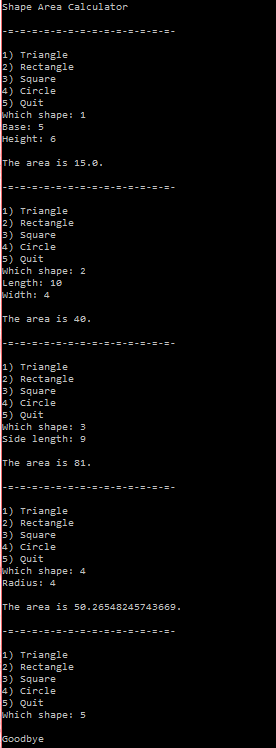# Assignemnt #97, Area Calculator

## Code

```/// Name: Alex Pinder
/// Period: 5
/// Program Name: Area Calculator
/// File Name: prog97.java
/// Date Complete: 6/4/16

import java.util.Scanner;

public class prog97
{
public static void main( String[] args )
{
Scanner keyboard = new Scanner(System.in);

System.out.println( "Shape Area Calculator" );

int ShapeNo = 0;
int x, y;

while ( ShapeNo != 5 )
{
System.out.print( "\n-=-=-=-=-=-=-=-=-=-=-=-=-=-=-\n\n1) Triangle\n2) Rectangle\n3) Square\n4) Circle\n5) Quit\nWhich shape: " );
ShapeNo = keyboard.nextInt();

if ( ShapeNo == 1 )
{
System.out.print( "Base: " );
x = keyboard.nextInt();
System.out.print( "Height: " );
y = keyboard.nextInt();

System.out.println( "\nThe area is " + areaTriangle( x, y ) + "." );
}

else if ( ShapeNo == 2 )
{
System.out.print( "Length: " );
x = keyboard.nextInt();
System.out.print( "Width: " );
y = keyboard.nextInt();

System.out.println( "\nThe area is " + areaRectangle( x, y ) + ".");
}

else if ( ShapeNo == 3 )
{
System.out.print( "Side length: " );
x = keyboard.nextInt();

System.out.println( "\nThe area is " + areaSquare( x ) + "." );
}

else if ( ShapeNo == 4 )
{
x = keyboard.nextInt();

System.out.println( "\nThe area is " + areaCircle( x ) + "." );
}

else if ( ShapeNo > 5 )
{
System.out.println( "ERROR" );
}
}

System.out.println( "\nGoodbye" );
}

public static double areaCircle( int radius )
{

return areaCircle;
}

public static int areaRectangle( int length, int width )
{
int areaRectangle = length * width;

return areaRectangle;
}

public static int areaSquare( int side )
{
int areaSquare = side * side;

return areaSquare;
}

public static double areaTriangle( int base, int height )
{
double areaTriangle = 0.5 * base * height;

return areaTriangle;
}
}

```

### Picture of the output